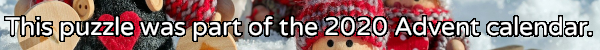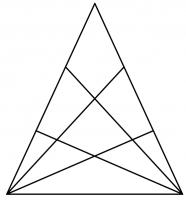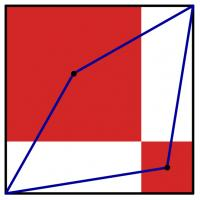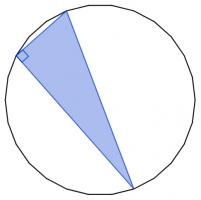mscroggs.co.uk
mscroggs.co.uksubscribe

# Puzzles

## 19 DecemberThe diagram to the right shows a triangle. Two of the sides of the triangle have been split into three pieces, with lines drawn from the opposite vertex. In total, the diagram now contains 27 triangles of any size.
Another triangle has two of its sides split into eight pieces, with lines drawn from the opposite vertex. How many triangles (of any size) would this create?

## 12 DecemberThe diagram to the left shows a large black square. Inside this square, two red squares have been drawn. (The sides of the red squares are parallel to the sides of the black square; each red square shares a vertex with the black square; and the two red squares share a vertex.) A blue quadrilateral has then been drawn with vertices at two corners of the black square and the centres of the red squares.
The area of the blue quadrilateral is 167. What is the area of the black square?

## 2 DecemberCarol draws a square with area 62. She then draws the smallest possible circle that this square is contained inside. Next, she draws the smallest possible square that her circle is contained inside. What is the area of her second square?

## 19 DecemberThe diagram below shows three squares and five circles. The four smaller circles are all the same size, and the red square's vertices are the centres of these circles.
The area of the blue square is 14 units. What is the area of the red square?

## 5 December28 points are spaced equally around the circumference of a circle. There are 3276 ways to pick three of these points. The three picked points can be connected to form a triangle. Today's number is the number of these triangles that are isosceles.

## 2 DecemberYou have 15 sticks of length 1cm, 2cm, ..., 15cm (one of each length). How many triangles can you make by picking three sticks and joining their ends?
Note: Three sticks (eg 1, 2 and 3) lying on top of each other does not count as a triangle.
Note: Rotations and reflections are counted as the same triangle.

## 23 DecemberToday's number is the area of the largest area rectangle with perimeter 46 and whose sides are all integer length.

## 12 DecemberThere are 2600 different ways to pick three vertices of a regular 26-sided shape. Sometimes the three vertices you pick form a right angled triangle.These three vertices form a right angled triangle.
Today's number is the number of different ways to pick three vertices of a regular 26-sided shape so that the three vertices make a right angled triangle.

## Archive

Show me a random puzzle
▼ show ▼